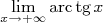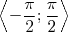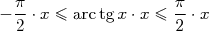# Limit of a function

how do you find the limit of this:this is not homework. i'm trying to learn how to do it.

EDIT:
and is there anything like 3 sequence theorems?

tiny-tim
Homework Helper
welcome to pf!

hi lalapnt! welcome to pf!how do you find the limit of this:

[PLAIN]http://www.matematyka.pl/latexrender/pictures/f/2/f2f7060d34f6c6a8b77a07d90e8c9b8f.png[/QUOTE] [Broken]

just draw the graph of tanx

then mark the positions of arctan100, arctan1000, etc …

where are they heading to?EDIT:
and is there anything like 3 sequence theorems?

sorry, not folloowing youLast edited by a moderator:
haruspex
Homework Helper
Gold Member
2020 Award
Are you asking what the answer is or how to prove it? You'll need to use the fact that the arc tangent function is defined to take the (principal) values (−π/2, π/2). Otherwise there will be multiple answers.

yes you're right. i need to know how to do such a question. and also the "domain" of the function isi want to know how to prove it. @haruspex

@tiny-tim do you have an idea what this is:Firstly, you have to know how to draw the graph of such funtions as (log, tang, cot, sin, cos...) then try to draw the inverse graph of these functions. You should also know about limit and its theorems. That's how I try to find a way to solve that kind of problems.
$\lim_{x\rightarrow+∞}arctgx =?$
When you draw the graph of arctgx and take the limit x goes to positive infinity, the function converges $\frac{\pi}{2}$. Eventually, we conclude the answer is
$\lim_{x\rightarrow+∞}arctgx = \frac{\pi}{2}$

Last edited by a moderator:
haruspex